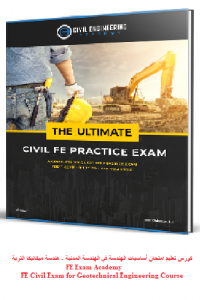اسم المؤلف
غير معروف
التاريخ
10 يناير 2023
المشاهدات
367
التقييم(لا توجد تقييمات)Loading...

كورس تعليم امتحان أساسيات الهندسة في الهندسة المدنية – هندسة ميكانيكا التربة
FE Exam Academy – FE Civil Exam for Geotechnical Engineering Course

1. Conclusion \ 1. Conclusion
2. Introduction \ 1. Introduction about FE Civil Exam & course contents
3. Stability of soil \ 1. Shear strength
4. Soil properties & classifications \ 1. Soil index properties & phase relations
5. Retaining structures \ 1. Soil vertical stress
6. Foundations \ 1. Types of foundations
7. Foundations \ 2. Bearing capacity
8. Soil properties & classifications \ 2. Field tests part-1 [Water content, relative density & relative compaction]
9. Stability of soil \ 2. Slopes stability
10. Retaining structures \ 2. Soil horizontal stress
11. Soil properties & classifications \ 3. Field tests part-2 [Sieve analysis]
12. Foundations \ 3. Soil consolidation
13. Stability of soil \ 3. Soil stabilization methods
14. Retaining structures \ 3. Stability of retaining structures
15. Soil properties & classifications \ 4. Field tests part-3 [SPT & Atterberg limits]
16. Retaining structures \ 5. Practice Exam answers (Part 1)
17. Foundations \ 5. Practice Exam answers (Part 1)
18. Stability of soil \ 5. Practice Exam answers (Part 1)
19. Soil properties & classifications \ 5. Soil classification as per USCS
20. Retaining structures \ 6. Practice Exam answers (Part 2)
21. Foundations \ 6. Practice Exam answers (Part 2)
22. Stability of soil \ 6. Practice Exam answers (Part 2)
23. Soil properties & classifications \ 6. Soil classification as per AASHTO
24. Retaining structures \ 7. Practice Exam answers (Part 3)
25. Foundations \ 7. Practice Exam answers (Part 3)
26. Soil properties & classifications \ 8. Practice Exam answers (Part 1)
27. Retaining structures \ 8. Practice Exam answers (Part 4)
28. Soil properties & classifications \ 9. Practice Exam answers (Part 2)
29. Soil properties & classifications \ 10. Practice Exam answers (Part 3)
30. Soil properties & classifications \ 11. Practice Exam answers (Part 4)
31. Soil properties & classifications \ 12. Practice Exam answers (Part 5)
32. Soil properties & classifications \ 13. Practice Exam answers (Part 6)
33. Soil properties & classifications \ 14. Practice Exam answers (Part 7)
34. Soil properties & classifications \ 15. Practice Exam answers (Part 8)
35. Soil properties & classifications \ 16. Practice Exam answers (Part 9)

كلمة سر فك الضغط : books-world.net